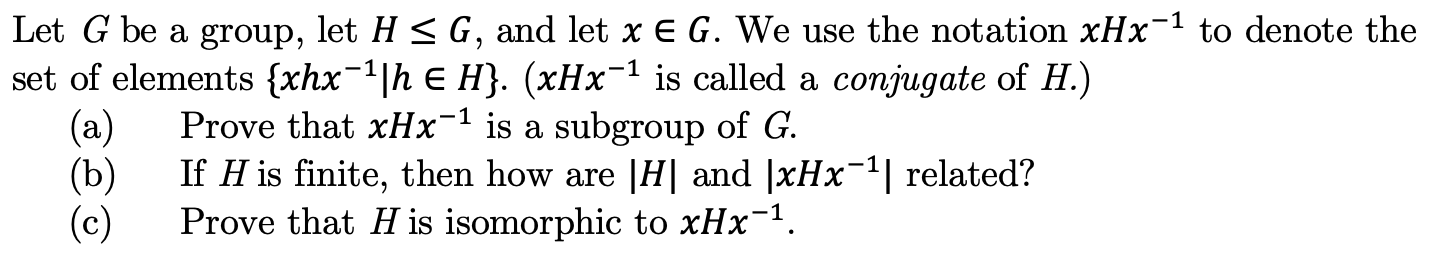Let G be a group, let H < G, and let x E G. We use the notation xHx1 to denote theset of elements {xhx1h E H}. (xHx is called a conjugate of H.)Prove that xHx-1 is a subgroup of G(a)If H is finite, then how are |H| and |xHx(b)(c)related?Prove that H is isomorphic to xHx1

Questionhelp_outlineImage TranscriptioncloseLet G be a group, let H < G, and let x E G. We use the notation xHx1 to denote the set of elements {xhx1h E H}. (xHx is called a conjugate of H.) Prove that xHx-1 is a subgroup of G (a) If H is finite, then how are |H| and |xHx (b) (c) related? Prove that H is isomorphic to xHx1 fullscreen
Step 1

In a group G, two element g and h are called conjugate when h = x g x-1 for some x G

For an element g of a group G, its conjugacy class is the set of elements conjugate to it:

{xgx−1 : x ∈ G}.

Part (a)          The set xHx-1 is a subgroup of G.

Proof: Since H is a subgroup, we know that H is nonempty, and therefore xHx-1 is nonempty.

Next,

let x h1 x-1 and x h2 x-1 be elements of xHx-1, where h1, h2 ∈ H.

Since H is a subgroup, we know that h1, h2 ∈ H, and therefore (x h1 x-1)( x h2 x-1  ) = xh1h2 x-1 is also an element of xHx-1.

Finally, let xhx-1 be any element of xHx-1 , where h ∈ H.

Since H is a subgroup, we know that h-1 ∈ H,

and therefore (x h1 x-1)-1= x h-1 x-1  is an element of xHx-1

Step 2

Part (b):

If H is a finite group, then each conjugacy class in H has size dividing |H|

Step 3

An isomorphism form H to xHx-1 is one-one mapping from H onto xHx-1 and preserve the group operation and xHx-1 is homomorphism:

First prove that...

Want to see the full answer?

See Solution

Want to see this answer and more?

Our solutions are written by experts, many with advanced degrees, and available 24/7

See Solution
Tagged in

Math